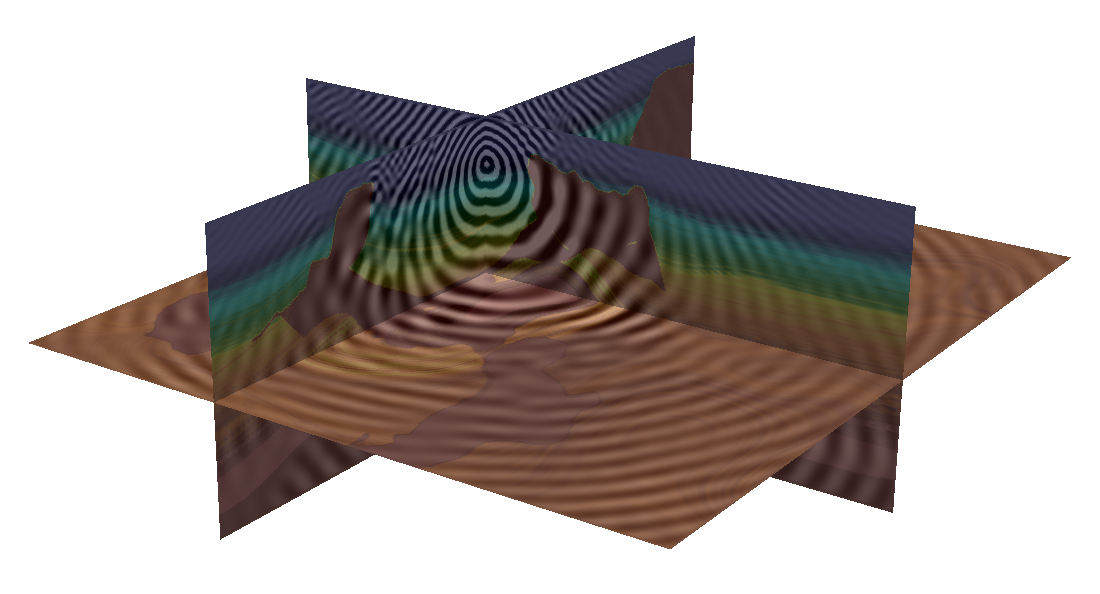### russell j. hewett

assistant professor

mathematics & CMDA# The method of polarized traces for the 3D Helmholtz equation

### Abstract

We present a fast solver for the 3D Helmholtz equation, in heterogeneous, constant density, acoustic media, in the high-frequency regime. The solver is based on the method of polarized traces, a layered domain-decomposition method, where the subdomains are connected via transmission conditions prescribed by the discrete Green’s representation formula and artificial reflections are avoided by enforcing non-reflecting boundary conditions between layers. The method of polarized traces allows us to consider only unknowns at the layer interfaces, reducing the overall cost and memory footprint of the solver. We demonstrate that polarizing the wavefields in this manner yields an efficient preconditioner for the reduced system, whose rate of convergence is independent of the problem frequency. The resulting preconditioned system is solved iteratively using GMRES, where we never assemble the reduced system or preconditioner, rather we implement them via solving the Helmholtz equation locally within the subdomains. The method is parallelized using MPI and coupled with a distributed linear algebra library and pipelining to obtain an empirical online runtime $\mathcal{O}(\max(1,R/L) N \log N)$ where $N = n^3$ is the total number of degrees of freedom, $L$ is the number of subdomains, and $R$ is the number of right-hand sides. This scaling is favorable for regimes in which the number of sources (distinct right-hand sides) is large, for example enabling large-scale implementations of frequency-domain full waveform inversion (FWI).

All publications
Publication
Geophysics